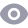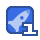### 数据结构与算法.pdf2收藏

void mainO play01(9); play02(9); 1ay03(9) system("pause"); } 13空间换时向思想 #includesiostream> # inc lude <map> using namespace std 计算一个由自然数1-1000组成的数组中,出现次数最多的数字 void play(int * array, int len) Int tmp={0};//事先分配—个内存空间用于缓存每个数出现的次数,在缓存的结果中求最大值 int max 0 for(inti=0;i<len;i+)//将出现次数放在下标位置-1处 int index= array[i]-1; tmp [i ndex++ for(inti=0;i< sizeof(tmp)/ sizeof(tmp);i++)//找出数组中出现最多的次数 if (max<tmp[i]) p[i]; for(inti=0;i< sizeof(tmp)/ sizeof(tmp);i++)//找到最多次对应的数字 if (max=tmp[i]) cout<<"出现最多次数的数字是:"<<i+1<<end1; void play02(int * array, int len, mapint, int> m) int max =0 feor(inti=0;i<len;i++)//将值和次数放进map中 pair<map<int, int>:: iterator, boo 1> pair1 =m insert(make_pair (array[i], 1)); if (pair. second==false) m[array[i] for (map<int, int>: iterator it=m. begin: it!=m endo; it++) if(max<(it->second)) max = it->second for (map<int, int>:: iterator it=m begin O; it != m endO; it++) if (max==(it->second)) cout <s it->first: vod main Int array[]={1,4,56,7,54,2,3,2,1,2,2,2,2,2,2,22,3,6,6,64,5,56,4,4,6,6,6,6}; int 1= sizeof carray)/ sizeof carray [o]) playcarray, 1); map<int, Int> playo2 (array, 1, m) system( pause"); 2线性表设计与实现 线性表的操作 创建线性表 销毁线性表 清空线性表 将元素插入线性表 将元素从线性表中删除 获取线性表中某个位置的元素 获取线性表的长度 2.1线性表顺序存储 21.1设计与实现 插入元素: 判断线性表是否合法 判断线性表容量是否已满 将插入位置后的所有元素后移(从最后一个元素开始) 插入元素到指定位置 线性表长度加1 获取元素: 判断线性表是否合法 判新位置是否合法 ·通过数组下标的方式获取元素 删除元素: 判断线性表是否合法 判新删除位置是否合法 将删除位置后的所有元素前移(从删除元素的下一个元素开始) 线性表长度减1 212案例实现(c) //seqlist. h #pragma once #includesiostream> using namespace std; typedef void seqlist typedef void seqlistNode; typedef struct tag_seqList int len; Int capacity; unsigned int *node; //int *node [] fTSeqlisti //创建线性表 Seqlist *seqListcreate (int capaci ty); //销毁线性表 void Seqlist_Destroy (SeqList "list); //清空链表 void Seqlist_clear(seqList *list) //链表长度 int seqlist_Length (seqlist *list) //链表容量 int SeqList_Capacity (seqlist * list) //将元素插入线性表 int SeqList_ Insert(SeqList *list, SeqListNode *node, int position) //获取线性表中某个位置的元素 seglistNode *seqlist_Get(seqlist *list, int position); //删除元素 Seglistnode seqList_Delete (seqlist *list, int position) //seqlist. cpp pragma once #includesiostream> using namespace std include"seglist h //创建链表 seqList *SeqList_Create(int capacity) int ret =0. TSegLlst“tmp=NULL; tmp- (Tseglist *)ma lloc(sizeof(tseglist)) if(tmp = NULL) et=-1 cout << func SeqList-CreateOerr :< ret < end1 return nUll memset(tmp, 0, sizeof(TSeqlist)); //根据 capaci ty分配节点空间 tmp->node (unsigned int *)mal loc(sizeof (unsigned int *)capacity) if (tmp->node== NULL) ret cout < "func Seglist Create err :" < ret < en return NULL tmp->capaci ty capacity, tmp->Ten=0 return tmp; //销毁链表 void seqlist Destroy (seqlist *list) TSeqList tlist = NULL; if (list = NULL) return tlist=(tseqlist *)list if (tlist->node ! NULL) free(tlist) //清空链表 void seqlist_clear(seqList *list) TSeqlist *tlist = NULL; if (list== NULL) return; tlist=(Tseqlist ")list: tlist->len= 0 //链表长度 int SeqList_Length (seqList *list) TSeqlist *tlist= NULL; if ( list = NULL) return -1 tlist=(TSeqlist s)list return tlist->len //链表容量 int seqList- capacity (seqlist *list) TSeqlist tlist =NULL if (list = NULL) return -1 tlist=(TSeqlist *)list: return tlist->capaci ty; //插入元素!!!!!!!!!!! int Seqlist_Insert (seqlist *list, seglistNode *node, int position) int ret =0 int i =0. TSeqList * tlist=NULL if (list== NULL node== NULL position< 0 ret =-1 cout << func SeqList_ InsertO err: < ret < end] return ret tlist= (tseqlist *)list: //判断容量是否已满 if(tlist->capacity < tlist->len) re 2 cout << func seqList_InsertO err (tlist->capacity < tlist->len) err :<< ret < endI return ret //容器修正,长度为6容量20用户插入位置为10 if (position > tlist->len) position= tlist->len //元素后移 for list->len; i position; i--) t1ist->node[i]= tlist->node[i-1];/ tlist-> node []为最后一个元素的下一个位置 //插入元素 tlist->node[i]= (unsigned int)node tlist->len++ seqlistNode *seqlist_Get(seqlist *list, int position) istNode ret 0 TSeglist *tlist=NULL f (i NULL positi cout <<func Seglist_Geto err < end I j return NULL i tlist =(tseqlist *)list ret= (void ")tlist->node [position] return ret //删除元素!!!!! seqlistNode* Seqlist_Delete (seqlist *list, int position) int i =0. SeqlistNode sret= 0 Seglist * tlist if (list = NULL position< 0) cout << func SeqList-DeleteOerr :" < endT return null tlist= (TSeqlist *)list: ret=(void *)tlist->node [posi tion //元素前移 for〔i= posltion;i<t1ist->len;i++)//从 posltIon位置后面的元素前移 tlist->node[i]= tlist->node[i +1] tlist->len-- return ret. //test. cpp include<iostream> using namespace std; #inc lude"seq list. h struct student student (int 1) age int age void maino Seglist *list=NULL student s1(10),s2(20),S3(30); list- SeqList_create(10) Seqlist_Capacity (list); Seqlist_Length(list) eqList_ Insert(list,( SeqListNode*)&s1,o);//头插法 Seqlist_ Insert(list, (seqlistNode *)&52, 0) Seglist_Insert(list, (seqlistNode *)&s3, 0) for (int i=0; i<seqList_Length (list); i++) tudent *t (student *)seglist_Get(list, i) if (tmp == NULL) { cout << student age:<< tmp->age < endT cout < d //删除链表中的头节点 st_Delete(list, o) for (int i=0; i<seqlist__ Length (list); i++) student *tmp =(student *)SeqList_Get (list, 1) if(tmp == NULL) return cout <<student age tmp->age nd1 seqlist_ Clear(1ist);/删除链表中的所有元素 Seqlist_ Destroy(list);//销毁链表 system( pause") 结果 tudent ag student age: 10 tudent age: 20 案例实现〔c++类模板) template<typename T> class SeqList bli SeqList(int capacity); seqlist o; int getlen(; int getcapaci ty (; int insert(t &t, int position) T&get〔 int position); T& del (int position); private: int len Int capacIty T* array;/数组 #include Seqlist. h temp late<typename t> Seqlist<T>:: SeqList(int capacity) parry new T[capacity]; this->capacity= capacity; this->len= 0 template<typename T> SeqList<t>:: seqListO if (this->array != NULL) delete[] array;//注意释放一个数组,需加[] array NULL, this->len =0: this->capaci ty =0; template<typename T> int SeqList<T>:: getlenO eturn this->len: template<typename T> int seqlist<T>: getcapacity o return this->capacity template<typename T> int seqList<T>:: insert(t &t, int position) int i= this->len; if (position 0) return -1 for(i;i> posltion;i--)//元素后移(从最后一个元素开始) parrayli]= parry [i-1]: array[i]=t;//stl元素保存时是通过复制的机制实现的,自己定义的类需要重载= 115->en++;

...展开详情

• 7
资源
• 31
粉丝
•等级

最新推荐数据结构与算法.pdf 50积分/C币 立即下载
1/98试读结束， 可继续阅读

50积分/C币 立即下载 ＞